#Function Repository Resource:

# ArcLine

A curved line between two points

Contributed by: Jon McLoone
 ResourceFunction["ArcLine"][{p1,p2},r] represents a curved 2D line segment joining points p1 and p2 with radius r. ResourceFunction["ArcLine"][{p1,p2},Scaled[n]] represents a curved 2D line segment joining points p1 and p2 with radius n times the distance between p1 and p2. ResourceFunction["ArcLine"][{p1,p2}] represents a curved 2D line segment joining points p1 and p2 with radius Scaled.

## Examples

### Basic Examples

Generate an arc between two points:

 In:=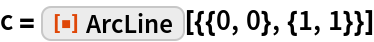Out=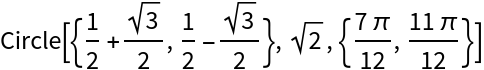Show this arc segment:

 In:=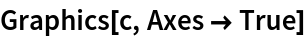Out=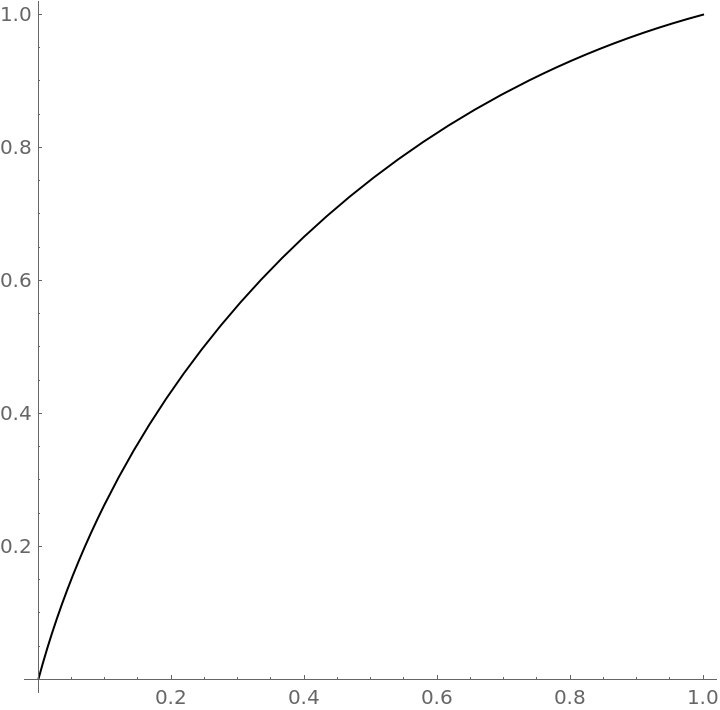### Scope

Arc radius can be set anywhere between the 1/2 of the Euclidean distance between the points and infinity. :

 In:=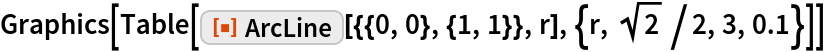Out=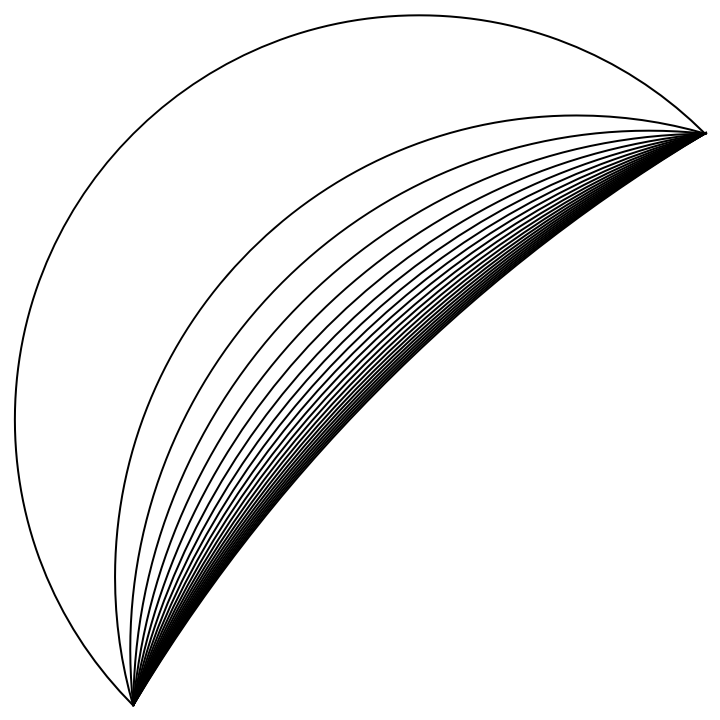Negative radius values change the orientation of the arc:

 In:=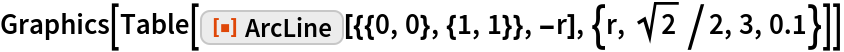Out=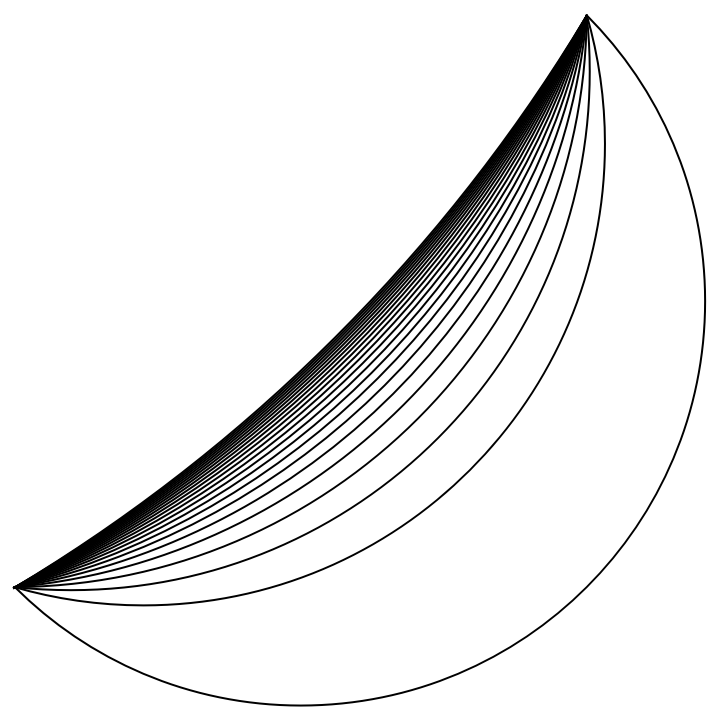Arc radius can be given as a multiple of EuclidianDistance between the points:

 In:=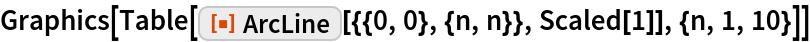Out=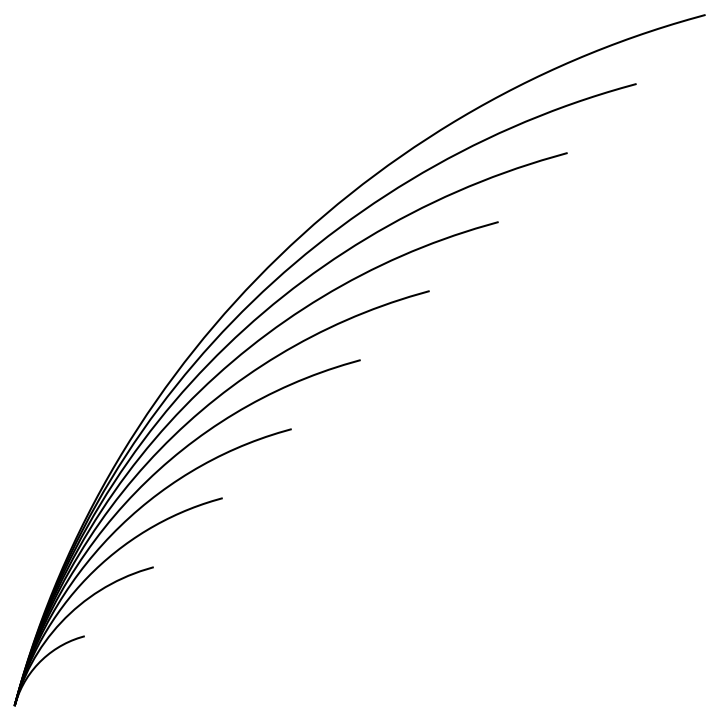### Properties and Relations

ArcLine with an Infinite radius is equivalent to Line:

 In:=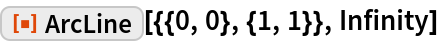Out=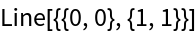### Possible Issues

The radius of the ArcLine cannot be less than 1/2 of the EuclideanDistance between the points:

 In:=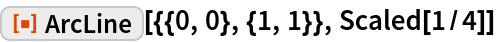Out=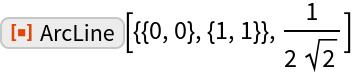In:=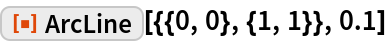Out=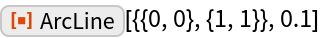### Neat Examples

You can create rounded regular polygons using CirclePoints:

 In:=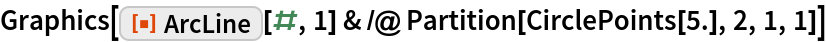Out=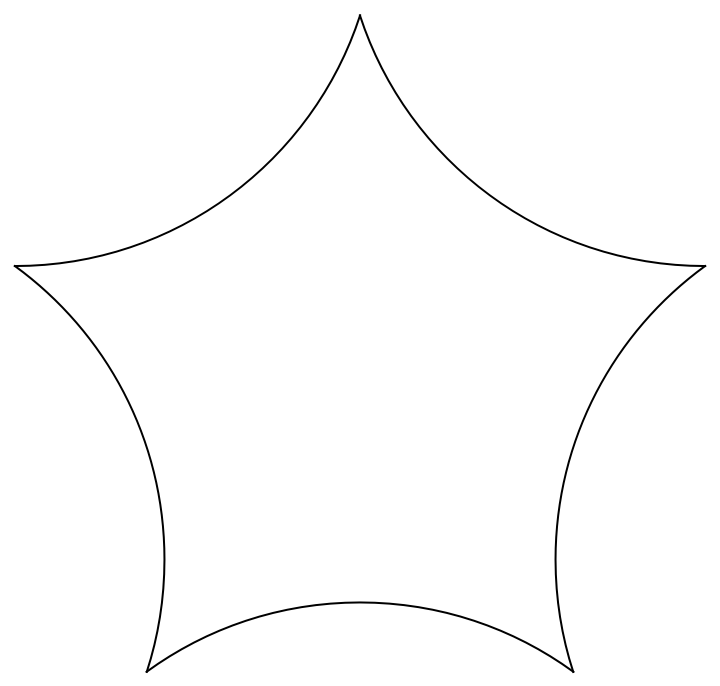In:=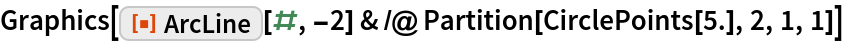Out=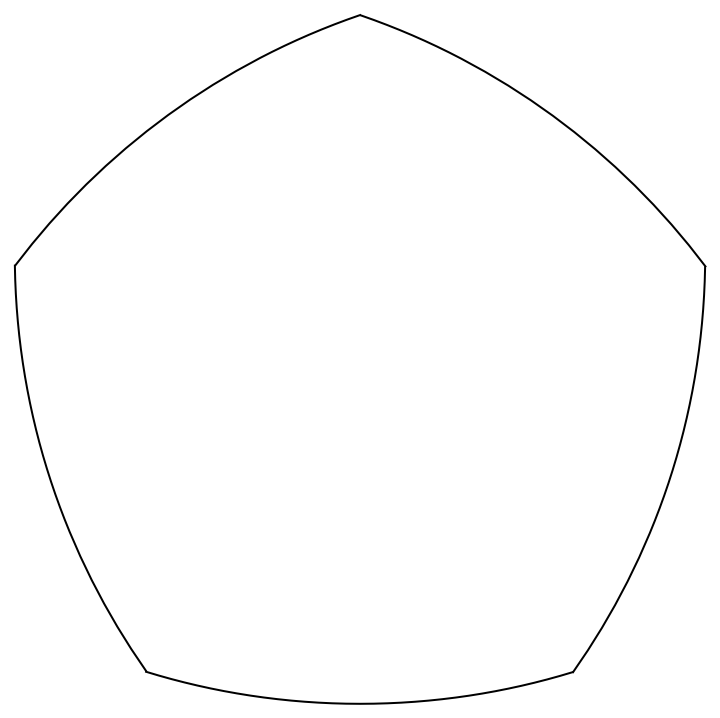## Requirements

Wolfram Language 11.3 (March 2018) or above